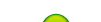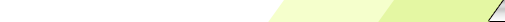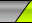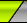Martes, 2023-10-03My siteMain | Registration | LoginWelcome Visitante | RSS
 Statistics Total en línea: 1 Invitados: 1 Usuarios: 0

# Anticovidian v.2 COVID-19: Hypothesis of the Lab Origin Versus a Zoonotic Event which can also be of a Lab Origin: https://zenodo.org/record/3988139

To go to previous page: http://fdocc.ucoz.com/index/0-88

# Einstein continues:

...We arrive at such a space, for example, in the following way. We start from a Euclidean space of four dimensions, ξ1, ξ2, ξ3, ξ4, with a linear element ds ; let, therefore,

ds 2 = 12 + 22 + 32 + 42 … (9)

In this space we consider the hyper-surface

R2 = ξ12 + ξ22 + ξ32 + ξ42 … (10)

The four-dimensional Euclidean space with which we started serves only for a convenient definition of our hyper-surface. Only those points of the hyper-surface are of interest to us which have metrical properties in agreement with those of physical space with a uniform distribution of matter. For the description of this three-dimensional continuum we may employ the co-ordinates ξ1, ξ2, ξ3 (the projection upon the hyper-plane ξ4 = 0) since, by reason of (10), ξ4 can be expressed in terms of ξ1, ξ2, ξ3. Eliminating ξ4 from (9) we obtain for the linear element of the spherical space the expression

ds 2 = gmn m n

... (11)

gmn = dmn + [(ξm ξn )/(R2r 2)]

where dmn = 1, if m = n ; dmn = 0, if mn , and r2 = ξ12 + ξ22 + ξ32. The coordinates chosen are convenient when it is a question of examining the environment of one of the two points ξ1 = ξ2 = ξ3 = 0.

Now the linear element of the required four-dimensional space-time universe is also given us. For the potential gmn, both indices of which differ from 4, we have to set

gmn = - (dmn + [(xm xn )/(R2 – (x12 + x22 + x32)] … (12)

which equation, in combination with (7) and (8), perfectly defines the behaviour of measuring-rods, clocks, and light-rays [(7) is: g44 = 1; (8) is: g14 = g24 = g34 = 0; whereas (4) is: m√- g gma(dxa/ds), but the text may be that it "differ from § 4” or that "differ from the number 4” as the number 4 is introduced in equations (2) and (15), see it below].

§ 4. On an Additional Term for the Field Equations of Gravitation

My proposed field equations of gravitation for any chosen system of co-ordinates runs as follows: -

Gmn = - k (Tmn – ½gmn T),

Gmn = -( /∂ xa ){mn, a} + {ma, b} {nb, a} + {[( 2log√-g)/(∂ xm xn )] - {mb, a}[( log√-g)/(∂ xma )}

The system of equations (13) is by no means satisfied when we insert for the gmn the values given in (7), (8), and (12), and for the (covariant) energy-tensor of matter the values indicated in (6). It will be shown in the next paragraph how this calculation may conveniently be made. So that, if it were certain that the field equations (13) which I have hitherto employed were the only ones compatible with the postulate of general relativity, we should probably have to conclude that the theory of relativity does not admit the hypothesis of a spatially finite universe.

[(6) is: 0 0 0 0

0 0 0 0

0 0 0 0 … (6) ]

0 0 0 r

However, the system of equations (14) allows a readily suggested extension (to admit the hypothesis of a spatially finite universe) which is compatible with the relativity postulate, and is perfectly analogous to the extension of Poisson’s equation given by equation (2). For on the left-hand side of field equation (13) we may add the fundamental tensor gmn , multiplied by a universal constant - l, at present unknown, without destroying the general covariance. In place of field equation (13) we write

Gmn = - l gmn = - k (Tmn – ½gmn T) … (13a)

This field equation, with l sufficiently small, is in any case also compatible with the facts of experience derived from the solar system. It also satisfies laws of conservation of momentum and energy, because we arrive at (13a) in place of (13) by introducing into Hamilton’s principle, instead of the scalar of Riemann’s tensor, this scalar increased by a universal constant ; and Hamilton’s principle, of course, guarantees the validity of laws of conservation. It will be shown in § 5 that field equation (13a) is compatible with our conjectures on field and matter [(2) is ▼2f - lf = 4pkr , where l denotes a universal constant].

§ 5 Calculation and Result

Since all points of our continuum are on an equal footing, it is sufficient to carry through the calculation for one point, e.g. for one of the two points with the co-ordinates

x1 = x2 = x3 = x4 = 0.

Then for the gmn in (13a) we have to insert the values

-1 0 0 0

0 -1 0 0

0 0 -1 0

0 0 0 1

wherever they appear differentiated only once or not at all. We thus obtain in the first place

Gmn = (∂ /∂ x1){mn, 1} + ( /∂ x2){mn, 2} + (∂ /∂ x3){mn, 3}+ [( 2log√-g)/(∂ xm xn)]

From this we readily discover, taking (7), (8), and (13) into account, that all equations (13a) are satisfied if the two relations

-(2/R2) + l = -(kr/2), - l = -(kr/2),

or

l = (kr/2) = (1/R2) … (14)

are fulfilled.

Thus the newly introduced universal constant l defines both the mean density of distribution r which can remain in equilibrium and also the radius R and the volume 2p2R3 of spherical space. The total mass M of the universe, according to our view, is finite, and is in fact

M = r . 2p2R3 = 4p2(R/k) = p2√(32/k3r) … (15)

Thus the theoretical view of the actual universe, if it is in correspondence with our reasoning, is the following. The curvature of space is variable in time and place, according to the distribution of matter, but we may roughly approximate to it by means of a spherical space. At any rate, this view is logically consistent, and from the standpoint of the general theory of relativity lies nearest at hand; whether, from the standpoint of present astronomical knowledge, it is tenable, will not here be discussed. In order to arrive at this consistent view, we admittedly had to introduce an extension of the field equations of gravitation which is not justified by our actual knowledge of gravitation. It is to be emphasized, however, that a positive curvature of space is given by our results, even if the supplementary term is not introduced. That term is necessary only for the purpose of making possible a quasi-static distribution of matter, as required by the fact of the small velocities of the stars.

---------------------------------

"Do Gravitational Fields Play An Essential Part In The Structure Of The Elementary Particles Of Matter" [Translated from "Spielen Gravitationsfelder im Aufber der materiellen Elementarteilchen eine wesentliche Rolle?", Sitzungsberichte der Preussischen Akademie der Wissenschaften, 1919]

To go to Einstein 5: http://fdocc.ucoz.com/index/0-90

 Search
 Site friends Create your own site